# Module 4 - Correlogram Analysis

In module one (1), we demonstrated the data preparation phase of time series analysis. In module two, we described a few steps to calculate numerous summary statistics and verify the significance of their values.

In this module, we present a few steps to conduct a correlogram analysis in Excel using NumXL functions and tools.

For sample data, we’ll use the S&P 500 closing logIn module 1, we showed that the logarithmic transformation of the prices provides better values distribution prices between January 2009 and July 2012.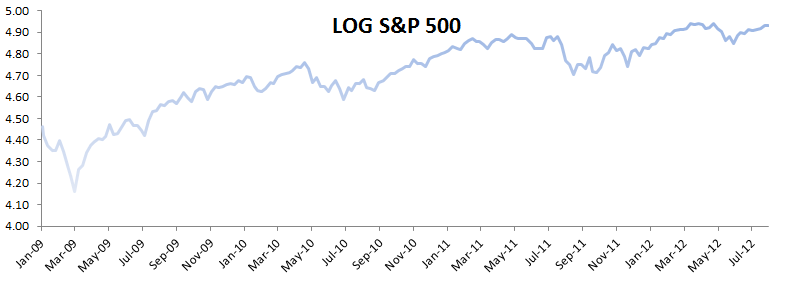Many time-series data sets exhibit time interdependency among their values. This is important to detect and will eventually factor in to improve the forecast quality of the model.

NumXL supports numerous functions and a wizard user interface, simplifying the process of constructing ACF and partial ACF (aka PACF) plots.

Using the NumXL Correlogram toolbar, you can generate the ACF/PACF values and their plots in a few steps.

1. Using the NumXL toolbar (or menu in Excel 97-2003), select Correlogram.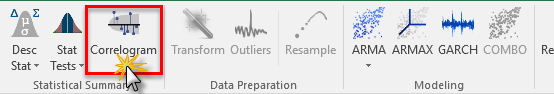2. The Correlogram dialog box pops up. Fill in the location of your data, series time order, output options and location for the table and graphs to be generated in your worksheet.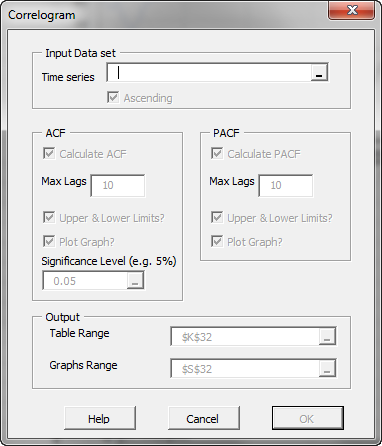3. Once finished, the tool prints out the table (along with the formulas) into the target cells and creates a correlogram plot (if selected).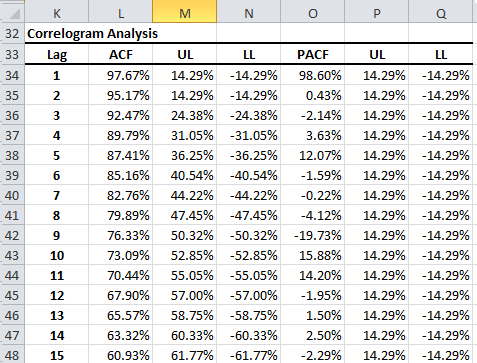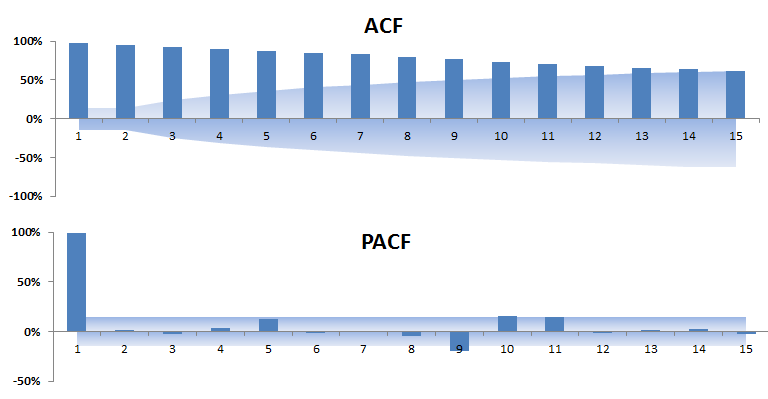The shaded area in the ACF and PACF plots represents the confidence intervals for the ACF and PACF values.

Note that PACF is significant (~100%) at lag order 1, and the ACF is declining very slowly. This is a common pattern indicating the presence of unit-rootIn module three, we tested the time series for the presence of unit-root..

Next, let’s take the first difference of the time series: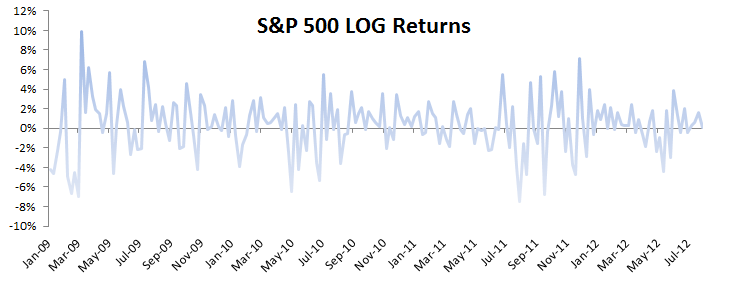Next, let’s run the correlogram analysis on the differenced (i.e. log returns) time series.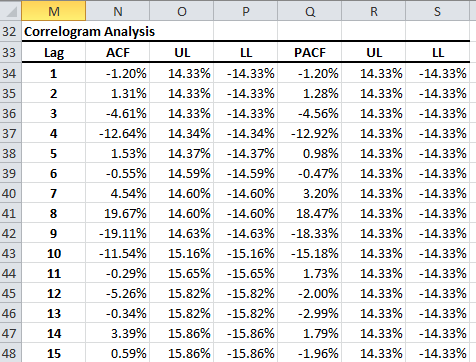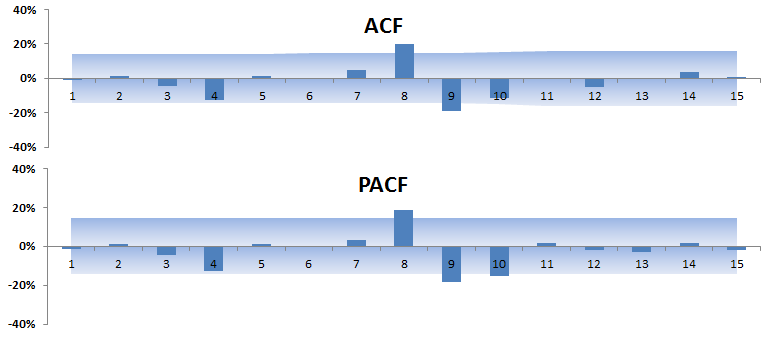The log returns do not exhibit strong interdependency, though lag order 8 and 9 show marginal significance. This beg the following question:

Q1: Does the log-returns time series exhibit white-noise (no serial correlation)?

To answer this question, we’ll use the descriptive statistics wizard and check the white-noise test option.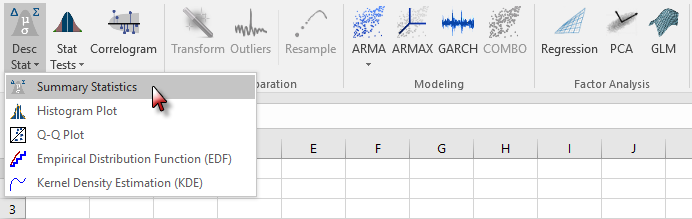Now, check the white-noise (Ljung-Box) test field:The summary statistics table with the white-noise test appears as follows:< p/>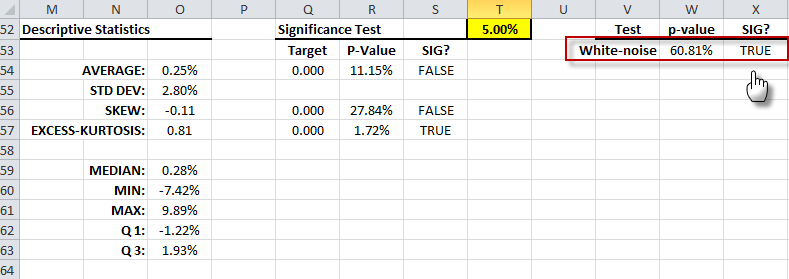The answer for our question is Yes, the time series does not exhibit significant serial correlation

### What's Next

The weekly log-returns time series distribution possesses a fat-tails (i.e. excess-kurtosis > 0), which may happen if the squared returns are correlated (aka ARCH effect).

Q: Does the log-returns time series exhibit an ARCH effect? Are the squared weekly log-returns correlated or more like a white-noise distribution?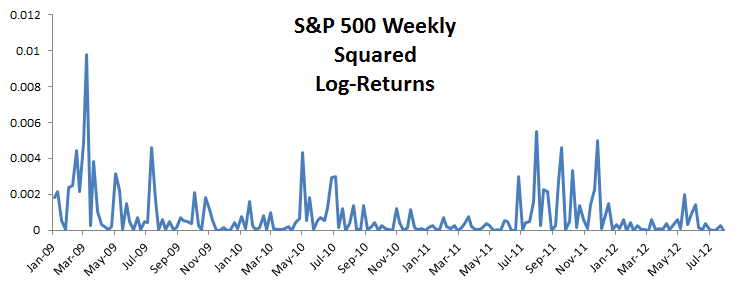Again, launch the descriptive statistics wizard from the NumXL toolbar (or menu in Excel 2003), and select the ARCH effect.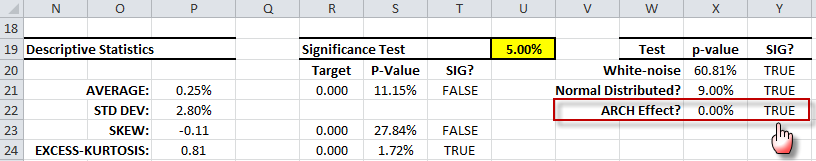Examining the the ARCH effect test results, we conclude that the squared returns are serially correlated; i.e. we have a conditional heteroskedacity in the log returns.

Let’s examine the correlogram of the squared log-returns: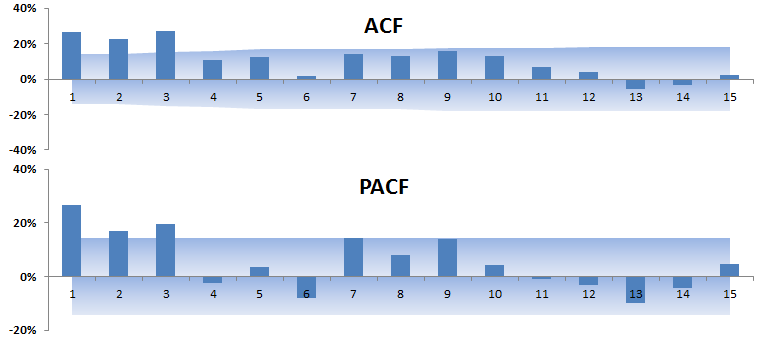The PACF shows a significant autocorrelation up to the 3rd lag order.

## Conclusion

The correlogram analysis is a key tool to explore the inter dependency of the observation values; it can also be used as a tool to identify the model and the estimate the orders of its components.

In our example, we found that the weekly log returns are not correlated, but their squared values are. As a result, an ARCH/GARCH model may be in order here.